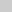Practice Test #1

1.How many bits are in 2 bytes?

2.A computer that uses vacuum tubes is newer than one that uses transistors.

3.What do you call a base-16 numbering system?

4.What is the sum of 1 and 1 in binary?

5.A byte can represent a decimal number between 0 and ?

6.What kind of program is used to convert source code to executable code?

7.What variable type would use the least memory to store a persons age?

8.What variable type would be best for storing the world's population?

 9.What will the last line of this code output? select hello Hello 10 hello 10 int Hello; cout << "Enter a value: "; cin >> Hello;                 //user enters "10" cout << "Hello";

 10.What will the last line of this code output? select 29 81 41 who knows!!!! int A, B; cout << "Enter a value: "; cin >> B;                     //user enters "5" cout << "Enter a value: "; cin >> A;                     //user enters "4" cout << A+B*A+B;

 11.What will this code output? select 6.5 5.5 7.5 8.5 float X = 5.5, Y = 0; X++; Y++; X = X + Y * 2; cout << X;

 12.What will this code output? select 0 1 2 3 short int Two = 1; short int Three = 2; short int Four = Two + Three; cout << Four % 2;

13.What's the largest number an unsigned short int variable be?

14.What's the smallest number an unsigned int variable can be?

15.What variable type would be best for storing the value of Pi?

You got out of correct. Your Score: %